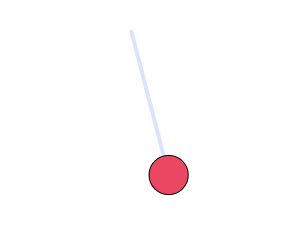# Physics Simulations

### The Three-Body ProblemThe three-body problem considers three or more massive objects that interact gravitationally according to Newton's Law of Gravitation. The problem cannot be solved analytically, and must be solved by computation.

### Single PendulumThis simulation considers a single pendulum moving under gravity. Rather than simply model the motion using a sine function, this simulation calculates the forces acting on the pendulum, and determines the changes to the kinematic variables based on those.